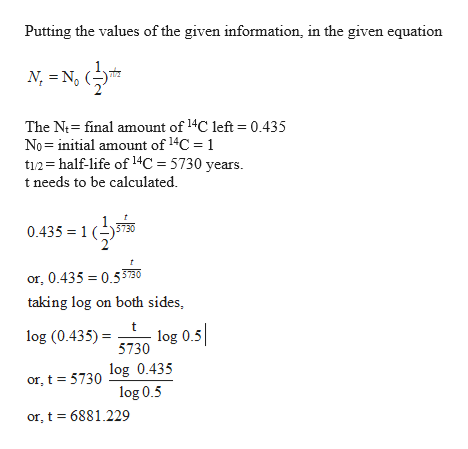Question

#2

Step 1

In this context, it is given that, the half-life (t1/2) of the 14C isotope is 5730 years. The researcher mentioned that the proportion of 14C found in Otzi’s body was 0.435 of the fraction usually found in a dead person’s body.

Now, it is also mentioned that when Otzi died, the amount of 14C present in Otzi’s body was 1.

Step 2help_outlineImage TranscriptionclosePutting the values of the given information, in the given equation N-N, The N final amount of14C left = 0.435 No initial amount of14C 1 ti half-life of14C = 5730 years t needs to be calculated 0.435 =1 3730 or, 0.435 0.530 taking log on both sides log (0.435)3730 log 0.5 or, t 5730 log 0.435 log 0.5 or, t 6881.229 fullscreen
Step 3

It has been mentioned that Otzi’s body was found at the end of 1991, thus, till 2019, 28 years have passed.

So, the final t = 6881.29 + 28 years = 6909.29 years.

Thus, it can be concluded tha...

Want to see the full answer?

See Solution

Want to see this answer and more?

Our solutions are written by experts, many with advanced degrees, and available 24/7

See Solution
Tagged in

Biology